Chapter 1 Class 7 Integers
Serial order wise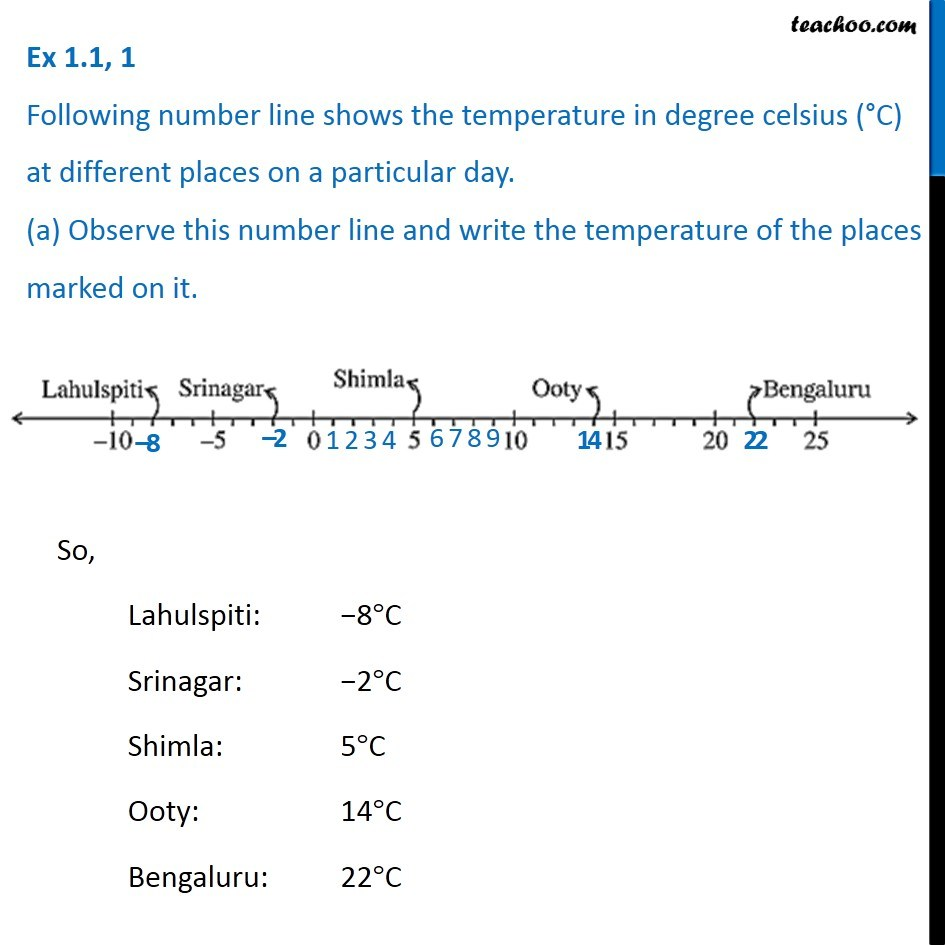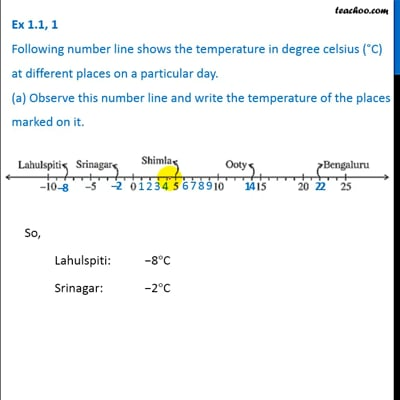This video is only available for Teachoo black users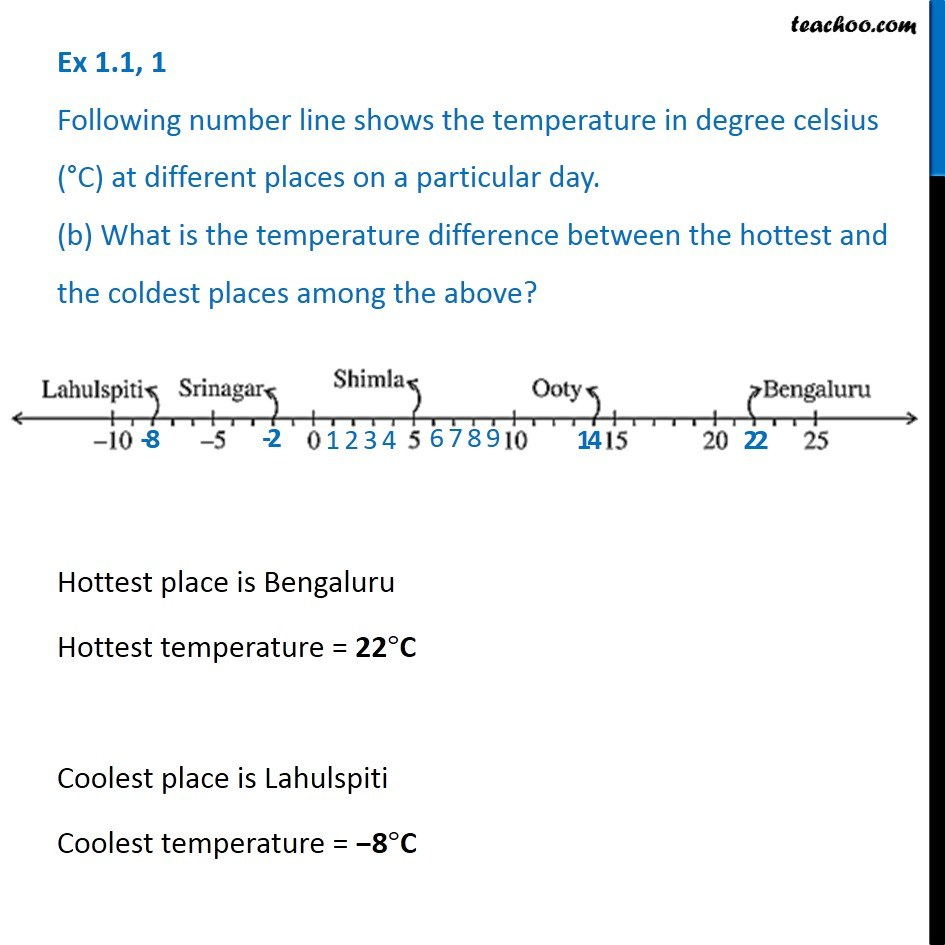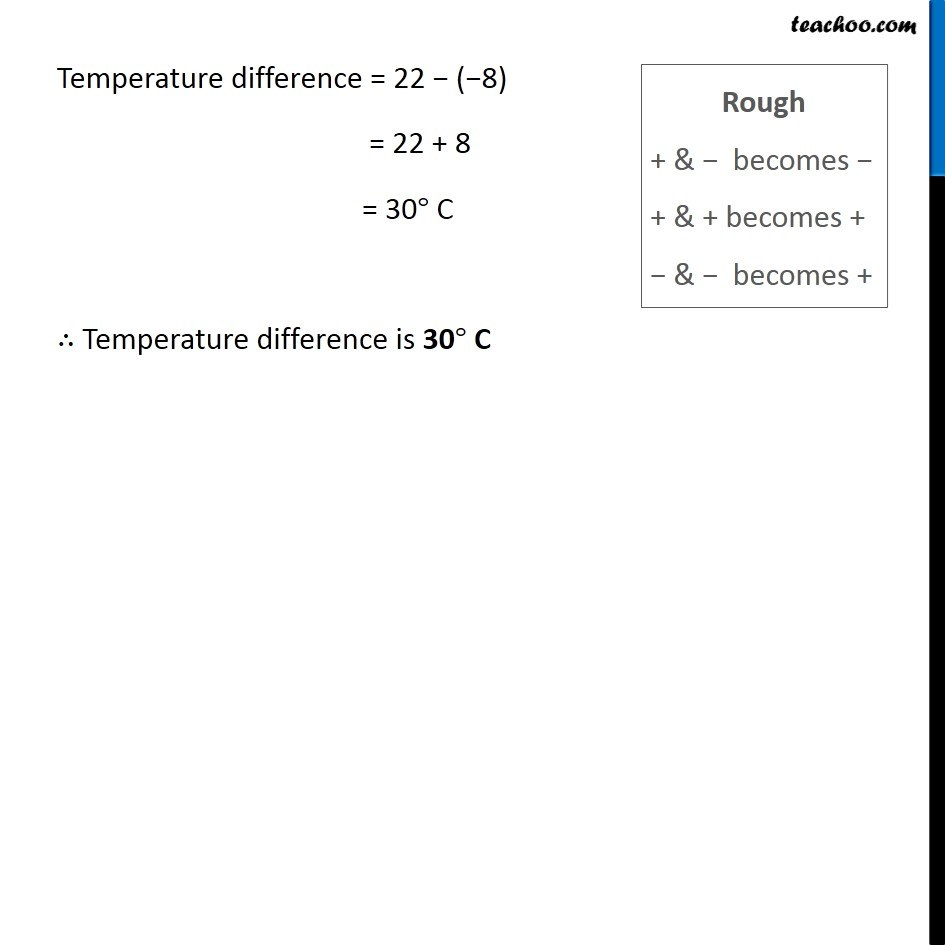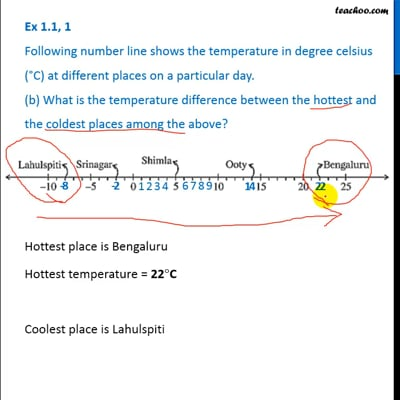This video is only available for Teachoo black users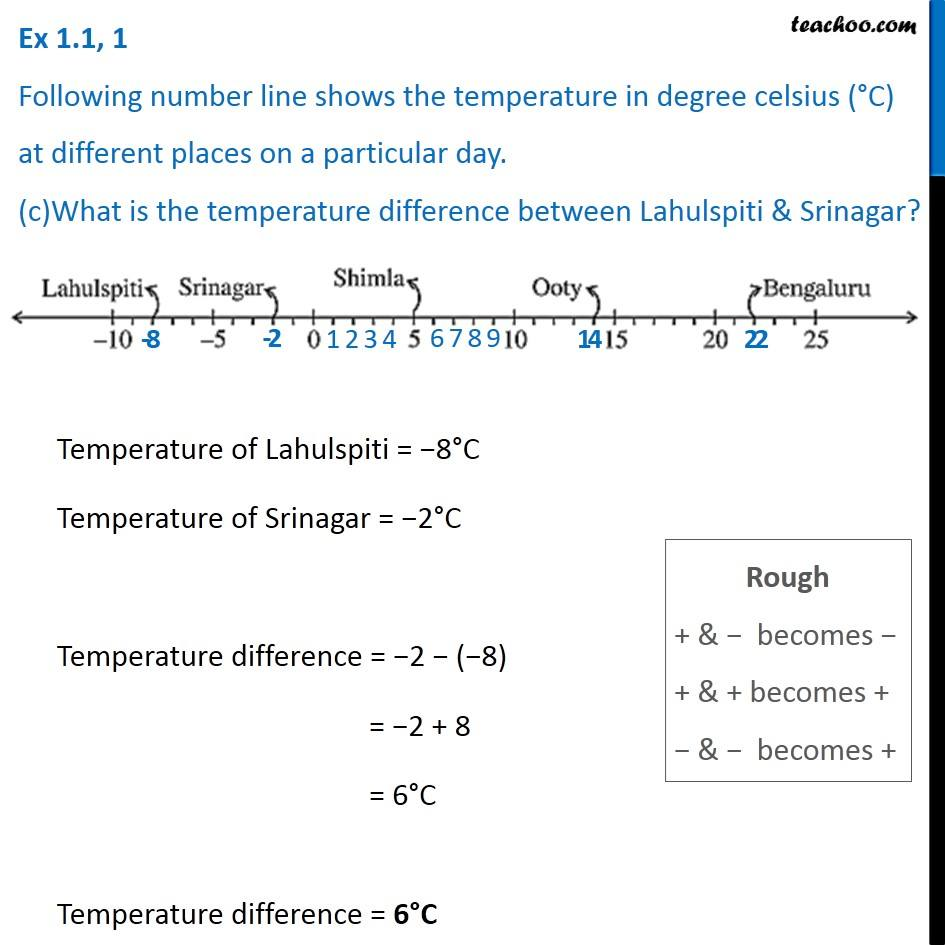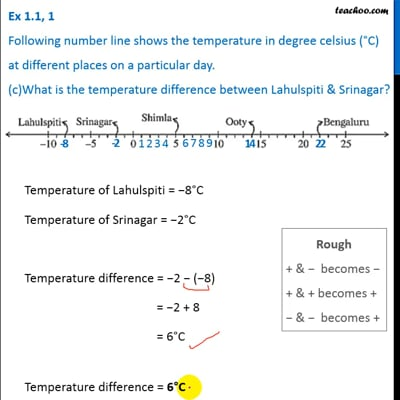This video is only available for Teachoo black users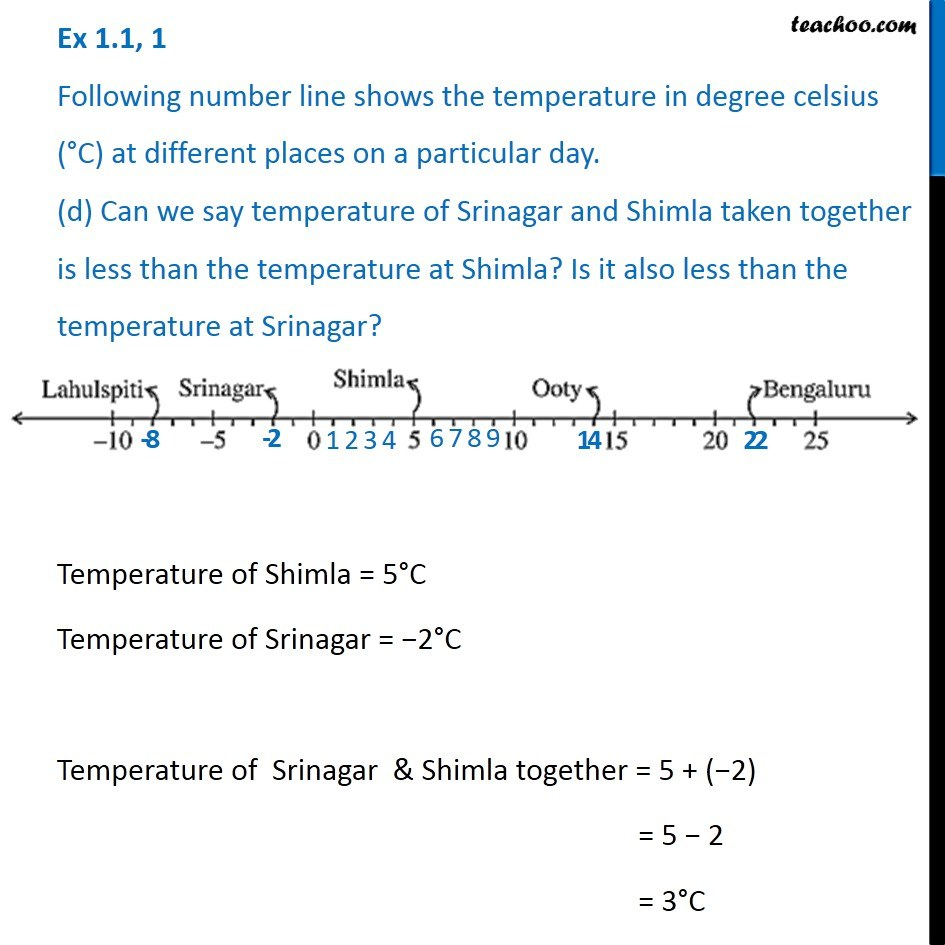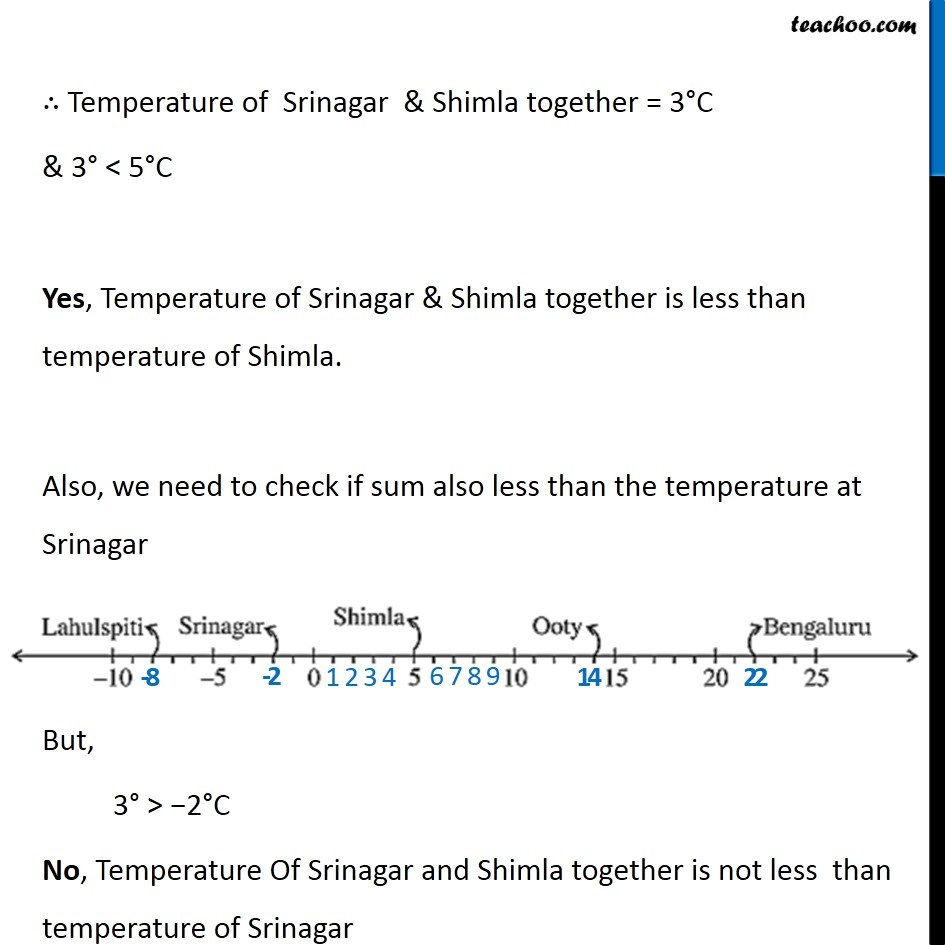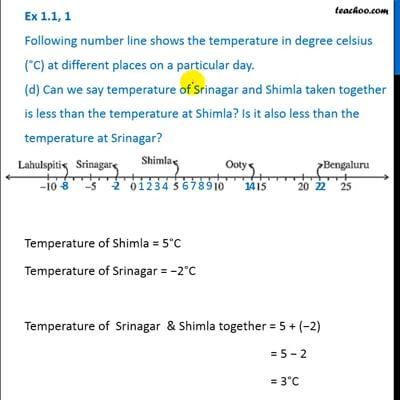This video is only available for Teachoo black users

Learn in your speed, with individual attention - Teachoo Maths 1-on-1 Class

### Transcript

Question 1 Following number line shows the temperature in degree celsius (°C) at different places on a particular day. (a) Observe this number line and write the temperature of the places marked on it. So, Lahulspiti: −8°C Srinagar: −2°C Shimla: 5°C Ooty: 14°C Bengaluru: 22°C Question 1 Following number line shows the temperature in degree celsius (°C) at different places on a particular day. (b) What is the temperature difference between the hottest and the coldest places among the above? Hottest place is Bengaluru Hottest temperature = 22°C Coolest place is Lahulspiti Coolest temperature = −8°C Temperature difference = 22 − (−8) = 22 + 8 = 30° C ∴ Temperature difference is 30° C Rough + & − becomes − + & + becomes + − & − becomes + Question 1 Following number line shows the temperature in degree celsius (°C) at different places on a particular day. (c)What is the temperature difference between Lahulspiti & Srinagar? Temperature of Lahulspiti = −8°C Temperature of Srinagar = −2°C Temperature difference = −2 − (−8) = −2 + 8 = 6°C Temperature difference = 6°C Rough + & − becomes − + & + becomes + − & − becomes + Question 1 Following number line shows the temperature in degree celsius (°C) at different places on a particular day. (d) Can we say temperature of Srinagar and Shimla taken together is less than the temperature at Shimla? Is it also less than the temperature at Srinagar? Temperature of Shimla = 5°C Temperature of Srinagar = −2°C Temperature of Srinagar & Shimla together = 5 + (−2) = 5 − 2 = 3°C ∴ Temperature of Srinagar & Shimla together = 3°C & 3° < 5°C Yes, Temperature of Srinagar & Shimla together is less than temperature of Shimla. Also, we need to check if sum also less than the temperature at Srinagar But, 3° > −2°C No, Temperature Of Srinagar and Shimla together is not less than temperature of Srinagar